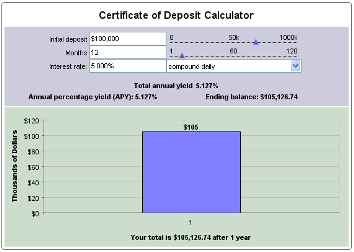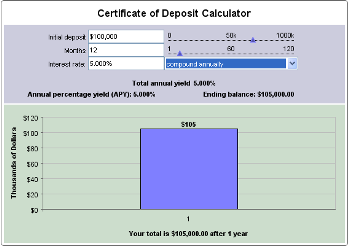Bitcoin tops 41,000. Check current cryptocurrency prices here: MonitorBankRates.com

# Using a CD Calculator

CD calculators are very easy to use and can be very helpful in figuring out how much interest you will earn on a certificate of deposit. To help you figure out the rate of return on your certificate of deposit investment all you have to do is enter the CD deposit amount, the term of the CD, the CD interest rate and how interest will be compounded, daily, weekly, monthly or annually.The more frequently interest is compounded the higher the overall return and annual percentage yield will be. Here is an example to give you a better idea.\$100,000 deposited in a 12 month CD with an interest rate of 5.00% compounded daily will have an APY of 5.127%. Earning \$5,126.74 in interest.\$100,000 deposited in a 12 month CD with an interest rate of 5.00% compounded annually will only have an APY of 5.00%. Earning \$5,000 in interest, \$126.74 less then a CD that has interest compounded daily.This is the power of compound interest, earning interest on top of interest. \$100,000 deposited in a 5 year CD with the same interest rate above compounded daily will earn \$28,400.31, compounded annually will only earn \$27,628.13, a difference of \$772.18.

Author: Brian McKay
December 25th, 2009
Posted in: CD Rates